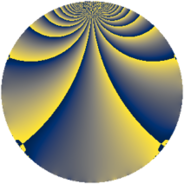# Properties

 Label 546.2.zLevel $546$ Weight $2$ Character orbit 546.z Rep. character $\chi_{546}(131,\cdot)$ Character field $\Q(\zeta_{6})$ Dimension $64$ Newform subspaces $2$ Sturm bound $224$ Trace bound $11$

# Related objects

## Defining parameters

 Level: $$N$$ $$=$$ $$546 = 2 \cdot 3 \cdot 7 \cdot 13$$ Weight: $$k$$ $$=$$ $$2$$ Character orbit: $$[\chi]$$ $$=$$ 546.z (of order $$6$$ and degree $$2$$) Character conductor: $$\operatorname{cond}(\chi)$$ $$=$$ $$21$$ Character field: $$\Q(\zeta_{6})$$ Newform subspaces: $$2$$ Sturm bound: $$224$$ Trace bound: $$11$$ Distinguishing $$T_p$$: $$5$$

## Dimensions

The following table gives the dimensions of various subspaces of $$M_{2}(546, [\chi])$$.

Total New Old
Modular forms 240 64 176
Cusp forms 208 64 144
Eisenstein series 32 0 32

## Trace form

 $$64q + 32q^{4} + 4q^{7} + 4q^{9} + O(q^{10})$$ $$64q + 32q^{4} + 4q^{7} + 4q^{9} + 12q^{10} - 24q^{15} - 32q^{16} - 8q^{21} - 24q^{22} - 12q^{24} - 36q^{25} - 4q^{28} + 2q^{30} - 12q^{31} + 8q^{36} + 12q^{40} - 4q^{42} + 64q^{43} + 32q^{46} - 52q^{49} - 2q^{51} - 16q^{57} - 20q^{58} - 12q^{60} + 72q^{61} + 56q^{63} - 64q^{64} + 48q^{66} - 36q^{70} - 96q^{73} + 12q^{75} + 36q^{79} - 4q^{81} - 16q^{84} - 64q^{85} - 12q^{87} - 12q^{88} - 16q^{91} + 52q^{93} - 12q^{96} + O(q^{100})$$

## Decomposition of $$S_{2}^{\mathrm{new}}(546, [\chi])$$ into newform subspaces

Label Dim. $$A$$ Field CM Traces $q$-expansion
$$a_2$$ $$a_3$$ $$a_5$$ $$a_7$$
546.2.z.a $$32$$ $$4.360$$ None $$0$$ $$0$$ $$0$$ $$2$$
546.2.z.b $$32$$ $$4.360$$ None $$0$$ $$0$$ $$0$$ $$2$$

## Decomposition of $$S_{2}^{\mathrm{old}}(546, [\chi])$$ into lower level spaces

$$S_{2}^{\mathrm{old}}(546, [\chi]) \cong$$ $$S_{2}^{\mathrm{new}}(21, [\chi])$$$$^{\oplus 4}$$$$\oplus$$$$S_{2}^{\mathrm{new}}(42, [\chi])$$$$^{\oplus 2}$$$$\oplus$$$$S_{2}^{\mathrm{new}}(273, [\chi])$$$$^{\oplus 2}$$